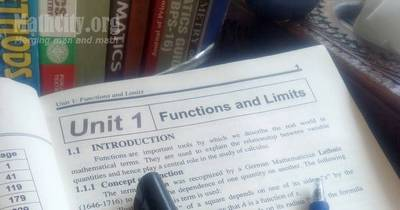Unit 01: Functions and LimitsNotes (Solutions) of Unit 01:Functions and Limits, Calculus and Analytic Geometry, MATHEMATICS 12 (Mathematics FSc Part 2 or HSSC-II), Punjab Text Book Board Lahore. You can view online or download PDF. To view PDF, you must have PDF Reader installed on your system and it can be downloaded from Software section.

Contents & summary

• Introduction
• Concept of Function
• Definition (Function-Domain-Range)
• Notation and Values of a Function
• Graphs of Algebraic functions
• Graph of Functions Defined Piece-Wise
• Types of Functions
• Algebraic Function
• Trigonometric Functions
• Inverse Trigonometric Functions
• Inverse Trigonometric Functions
• Exponential Function
• Logarithmic Function
• Hyperbolic Function
• Inverse Hyperbolic Function
• Explicit Function
• Even Function
• Odd Function
• Exercise 1.1
• Composition of Function and Inverse of a Function
• Composition of Functions Explanation
• Inverse of a Function
• Algebraic Method to find the Inverse Function
• Exercise 1.2
• Limits of a Function and Theorems on Limits
• Meaning of the phrase “x approaches Zero”
• Meaning of the Phrase “x approaches a”
• Concept of Limit of a Function
• Limit of Function
• Theorems on Limits of Function
• Limits of Important Functions
• $\lim_{x\to a}\frac{x^n-a^n}{x-a} = na^{n-1}$, where n is an integer and a>0
• $\lim_{x\to0}\frac{\sqrt{x+a} - \sqrt{a}}{x} = \frac{1}{2\sqrt{a}}$
• Limit at Infinity
• Methods for Evaluating the limits at Infinity
• $\lim_{x\to0}(1+\frac{1}{n})^n = e$
• $\lim_{x\to0}\frac{a^x-1}{x} = {\log_e}^a$
• The Sandwitch theorem
• If $\theta$ is measured in radian, then $\lim_{\theta\to 0}\frac{\sin\theta}{\theta} = 1$
• Exercise 1.3
• Continuous and Discontinuous Function
• One-sided Limits
• Criterion for Existence of Limit of a Function
• Continuity of a function at a number
• Exercise 1.4
• Graphs
• Graph of the Exponential Function $f(x) = a^x$
• Graph of the Exponential Function $f(x) = e^x$
• Graph of Common Logarithmic Function $f(x) = \log x$
• Graph of natural logarithmic Function $f(x) = \ln x$
• Graph of Implicit Function
• Graph of parametric Equations
• Graph of Discontinuous Function
• Graphical Solution of the Equations
• Exercise 1.5
Did you know?
Consider two functions $f(x)=x+3$ and $\displaystyle g(x)=\frac{x^2-9}{x-3}$. Is $f=g$? (see answer here)